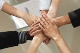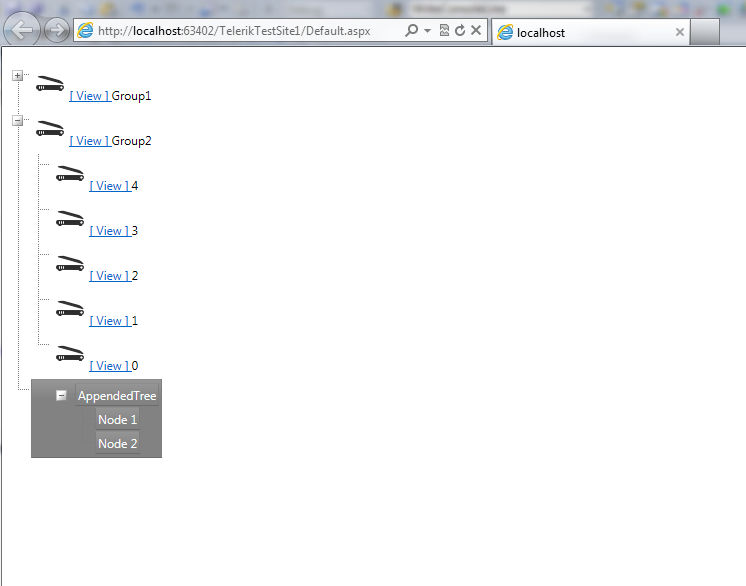# nesting treeView programatically

TreeViewTop achievementsRank 1
Aarsh asked on 20 Jul 2012, 03:46 AM
right now I have following setupand the code behind is :

`RadTreeNode category1 = ``new` `RadTreeNode(``"Group1"``, ``"Group1"``);`
`  ``RadTreeNode Category1Nodes;`
`  ``RadTreeNodeCollection radCollection = ``new` `RadTreeNodeCollection(category1);`
`  ``for` `(``int` `i = 10 - 1; i >= 0; i--)`
`  ``{`
`      ``Category1Nodes = ``new` `RadTreeNode(i.ToString(), i.ToString());`
`      ``category1.Nodes.Add(Category1Nodes);`
`  ``}`
`  ``RadTreeView1.Nodes.Add(category1);`
`      `

`  ``RadTreeNode category2 = ``new` `RadTreeNode(``"Group2"``, ``"Group2"``);`
`  ``RadTreeNode Category2Nodes;`
`  ``radCollection = ``new` `RadTreeNodeCollection(category2);`
`  ``for` `(``int` `i = 5 - 1; i >= 0; i--)`
`  ``{`
`      ``Category2Nodes = ``new` `RadTreeNode(i.ToString(), i.ToString());`
`      ``category2.Nodes.Add(Category2Nodes);`
`  ``}`
`  ``RadTreeView1.Nodes.Add(category2);`

now, I want to add Category3, but I am wondering if I can attach a treeView to that

Thanks,
-Aarsh

## 3 Answers, 1 is accepted

0Princy
Top achievementsRank 2
answered on 20 Jul 2012, 05:01 AM
Hi Aarsh,

C#:
`RadTreeNode category3 = ``new` `RadTreeNode();`
`protected` `override` `void` `OnInit(EventArgs e)`
` ``{`
`   ``category3.NodeTemplate = ``new` `TreeviewTemplate();`
`   ``base``.OnInit(e);`
` ``}`
`protected` `void` `Page_Load(``object` `sender, EventArgs e)`
` ``{`
`   ``RadTreeNode category1 = ``new` `RadTreeNode(``"Group1"``, ``"Group1"``);`
`   ``RadTreeNode Category1Nodes;`
`   ``RadTreeNodeCollection radCollection = ``new` `RadTreeNodeCollection(category1);`
`   ``for` `(``int` `i = 10 - 1; i >= 0; i--)`
`    ``{`
`      ``Category1Nodes = ``new` `RadTreeNode(i.ToString(), i.ToString());`
`      ``category1.Nodes.Add(Category1Nodes);`
`    ``}`
`   ``RadTreeView1.Nodes.Add(category1);`
`   ``RadTreeNode category2 = ``new` `RadTreeNode(``"Group2"``, ``"Group2"``);`
`   ``RadTreeNode Category2Nodes;`
`   ``radCollection = ``new` `RadTreeNodeCollection(category2);`
`   ``for` `(``int` `i = 5 - 1; i >= 0; i--)`
`    ``{`
`      ``Category2Nodes = ``new` `RadTreeNode(i.ToString(), i.ToString());`
`      ``category2.Nodes.Add(Category2Nodes);`
`    ``}`
`   ``RadTreeView1.Nodes.Add(category2);`
`   ``RadTreeView1.Nodes.Add(category3);`
` ``}`

`//For creating NodeTemplate`

`class` `TreeviewTemplate : ITemplate`
` ``{`
`   ``public` `void` `InstantiateIn(Control container)`
`    ``{`
`      ``RadTreeView RadTreeView2 = ``new` `RadTreeView();`
`      ``RadTreeView2.ID = ``"RadTreeView2"``;`
`      ``RadTreeNode node1 = ``new` `RadTreeNode();`
`      ``node1.Text = ``"node1"``;`
`      ``RadTreeView2.Nodes.Add(node1);`
`      ``RadTreeNode node2 = ``new` `RadTreeNode();`
`      ``node2.Text = ``"node2"``;`
`      ``RadTreeView2.Nodes.Add(node2);`
`      ``container.Controls.Add(RadTreeView2);`
`    ``}`
` ``}`

Hope this helps.

Thanks,
Princy.
0Aarsh
Top achievementsRank 1
answered on 20 Jul 2012, 12:50 PM
Awesome, that works :-)`RadTreeNode category3 = ``new` `RadTreeNode();`
`    ``protected` `override` `void` `OnInit(EventArgs e)`
`    ``{`
`        ``category3.NodeTemplate = ``new` `TreeViewTemplate();`
`    ``}`

`public` `class` `TreeViewTemplate : ITemplate`
`{`
`    ``public` `void` `InstantiateIn(Control container)`
`    ``{`
`        ``RadTreeView addRadTree = ``new` `RadTreeView();`
`        ``addRadTree.ID = ``"ProceedingTreeView"``;`

`        ``RadTreeNode SubHead = ``new` `RadTreeNode(``"AppendedTree"``, ``"AppendedTree"``);`
`        ``RadTreeNodeCollection nodesCollection = ``new` `RadTreeNodeCollection(SubHead);`

`        ``RadTreeNode node1 = ``new` `RadTreeNode(``"Node 1"``, ``"Node 1"``);`
`        ``SubHead.Nodes.Add(node1);`

`        ``RadTreeNode node2 = ``new` `RadTreeNode(``"Node 2"``, ``"Node 2"``);`
`        ``SubHead.Nodes.Add(node2);`

`        ``addRadTree.Nodes.Add(SubHead);`
`        ``addRadTree.DataBind();`

`        ``container.Controls.Add(addRadTree);`
`        `
`    ``}`
`}`

and in the main method, the treeview is being added in the following way ..

`RadTreeView1.Nodes.Add(category3);`

Now...

How can I add attributes to the "Appended Tree" tree ? I mean, I'd love to have following attributes in that tree :

1. Each node should be having an asp:ImageButton and NodeText. and we can assign  distinct links to imageButton as well, as the text.
2. Most importantly, the links should be assigned dynamically, [ as it would come from the data-layer, as per user's query hence, ] I want to get it assigned from within C#.

-Aarsh
0Princy
Top achievementsRank 2
answered on 23 Jul 2012, 06:44 AM
Hi Aarsh,

C#:
`RadTreeNode node = (RadTreeNode)RadTreeView1.FindNodeByText(``"category3"``);`
`RadTreeView addRadTree = (RadTreeView)node.FindControl(``"ProceedingTreeView"``);`
`RadTreeNode SubHead = addRadTree.FindNodeByText(``"AppendedTree"``);`
`ImageButton btn = (ImageButton)SubHead.FindControl(``"btn"``);`
`LinkButton lnkbtn = (LinkButton)SubHead.FindControl(``"lnkbtn"``);`
`btn.PostBackUrl = ``"Your link"``;`
`lnkbtn.PostBackUrl = ``"Your link"``;`
`RadTreeNode Node1 = addRadTree.FindNodeByText(``"Node 1"``);`
`ImageButton btn1 = (ImageButton)Node1.FindControl(``"btn"``);`
`LinkButton lnkbtn1 = (LinkButton)Node1.FindControl(``"lnkbtn"``);`
`btn1.PostBackUrl = ``"Your link"``;`
`lnkbtn1.PostBackUrl = ``"Your link"``;`
`RadTreeNode Node2 = addRadTree.FindNodeByText(``"Node 2"``);`
`ImageButton btn2 = (ImageButton)Node2.FindControl(``"btn"``);`
`LinkButton lnkbtn2 = (LinkButton)Node2.FindControl(``"lnkbtn"``);`
`btn2.PostBackUrl = ``"Your link"``;`
`lnkbtn2.PostBackUrl = ``"Your link"``;`

`class` `TreeviewTemplate : ITemplate`
`{`
`    ``public` `void` `InstantiateIn(Control container)`
`    ``{`
`      ``RadTreeView addRadTree = ``new` `RadTreeView();`
`        ``addRadTree.ID = ``"ProceedingTreeView"``;`
`        ``RadTreeNode SubHead = ``new` `RadTreeNode(``"AppendedTree"``, ``"AppendedTree"``);`
`        ``RadTreeNodeCollection nodesCollection = ``new` `RadTreeNodeCollection(SubHead);`
`        ``RadTreeNode node1 = ``new` `RadTreeNode(``"Node 1"``, ``"Node 1"``);`
`        ``SubHead.Nodes.Add(node1);`
`        ``RadTreeNode node2 = ``new` `RadTreeNode(``"Node 2"``, ``"Node 2"``);`
`        ``SubHead.Nodes.Add(node2);`
`        ``addRadTree.Nodes.Add(SubHead);`
`        ``Button template = ``new` `Button();`
`        ``template.InstantiateIn(SubHead);`
`        ``template.InstantiateIn(node1);`
`        ``template.InstantiateIn(node2);`
`        ``addRadTree.DataBind();`
`        ``container.Controls.Add(addRadTree);`
`    ``}`
`}`

`//NodeTemplate for addRadTree `

`class` `Button : ITemplate`
`{`
`    ``public` `void` `InstantiateIn(Control container)`
`    ``{`
`        ``ImageButton btn = ``new` `ImageButton();`
`        ``btn.ID = ``"btn"``;`
`        ``btn.ImageUrl = ``"~/Images/bullet5.jpg"``;`
`        ``container.Controls.Add(btn);`
`        ``LinkButton lnkbtn = ``new` `LinkButton();`
`        ``lnkbtn.ID = ``"lnkbtn"``;`
`        ``lnkbtn.Text = ``"Node"``;`
`        ``container.Controls.Add(lnkbtn);`
`    ``}`
`}`

Hope this helps.

Regards,
Princy.
Tags
TreeViewAarsh
Top achievementsRank 1Rank 2Rank 1